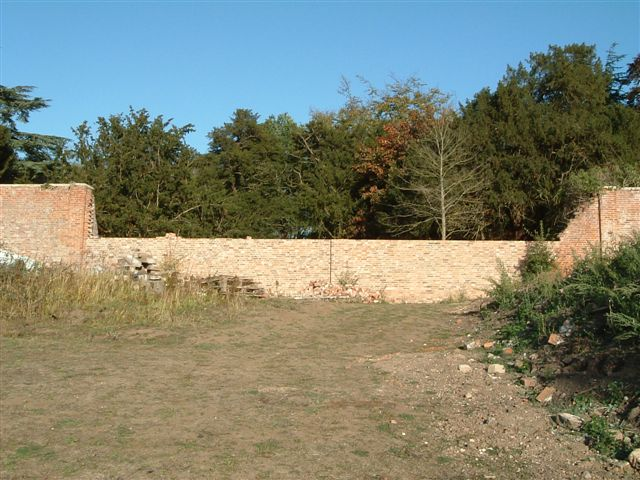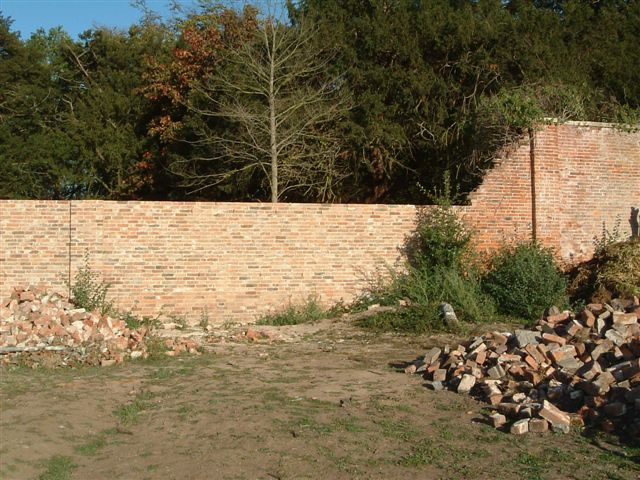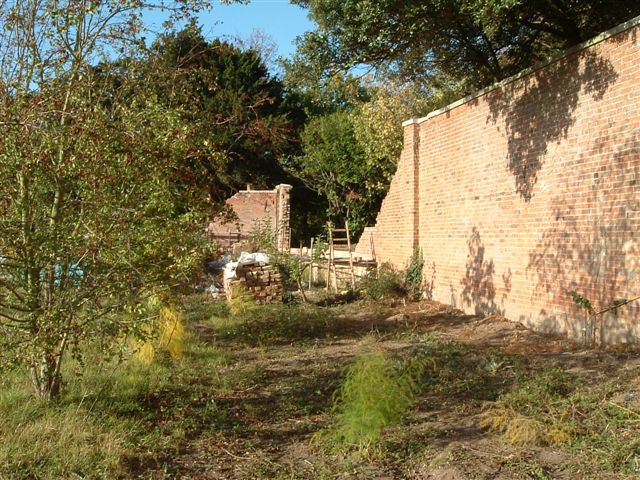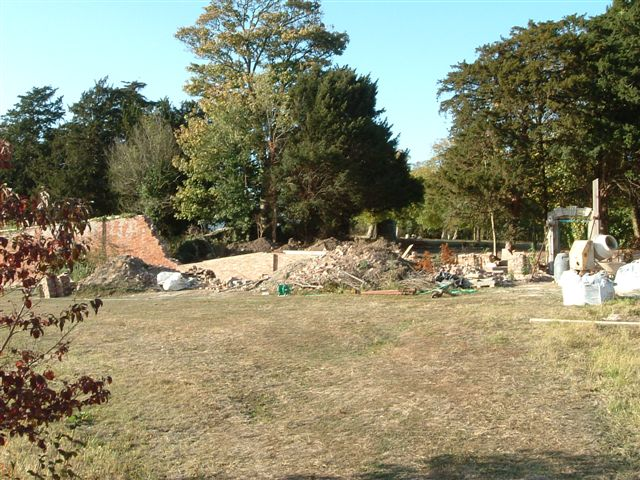## Momentum

A simple equation.  (P1 + P2 + P3) / T  = M {where P1 = preparation, P2 = persistence, P3 = pressure and T = time, the universal common denominator}. In other words, the walls are coming on in leaps and bounds.